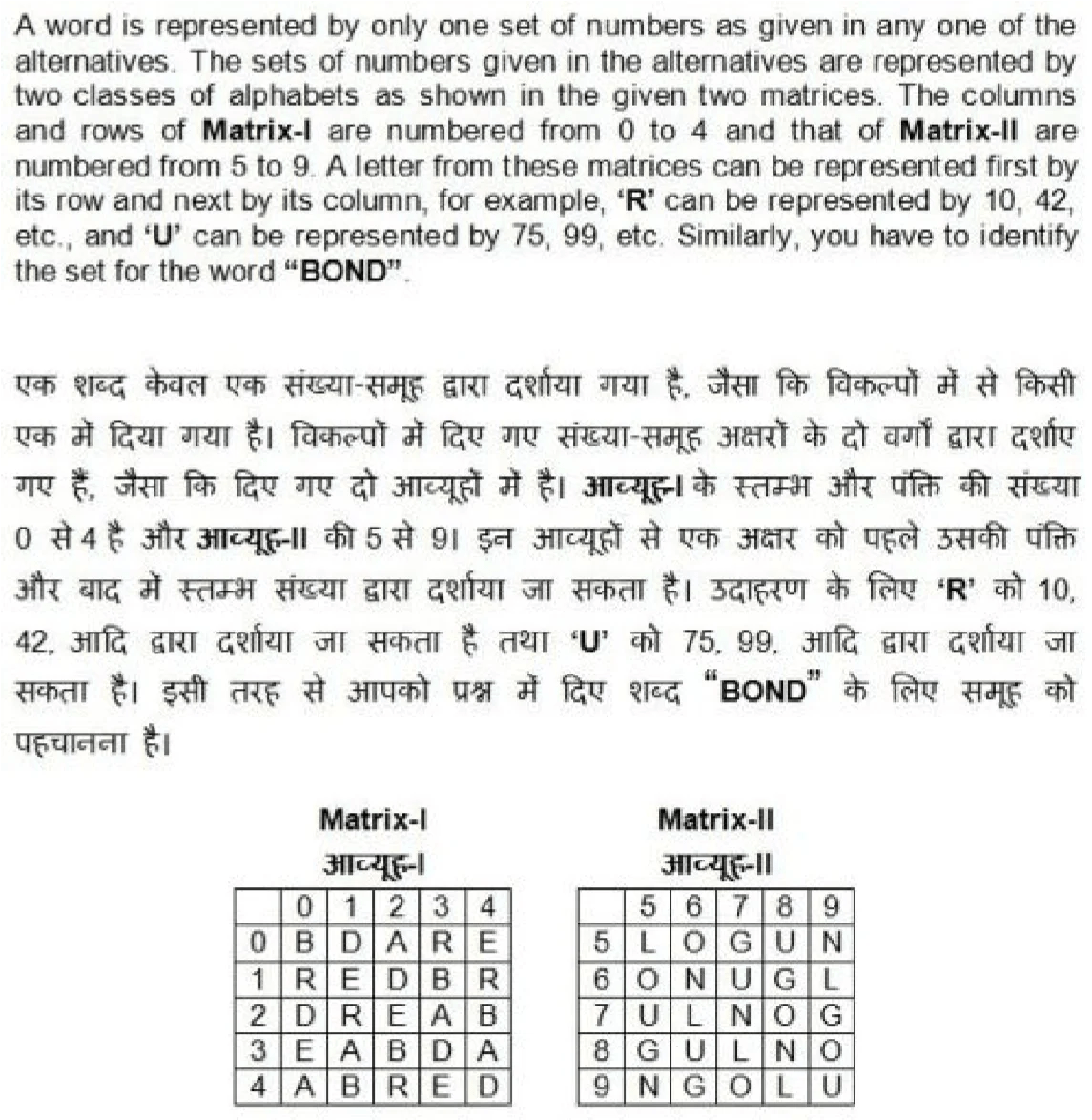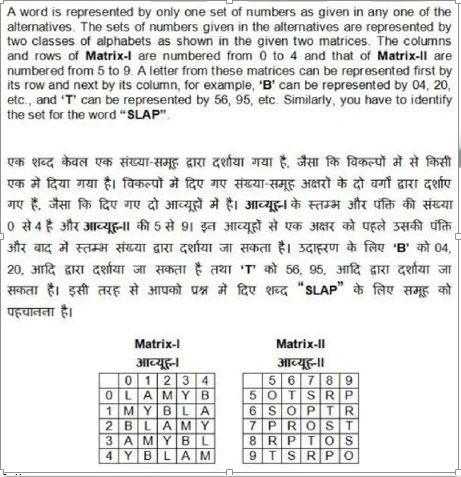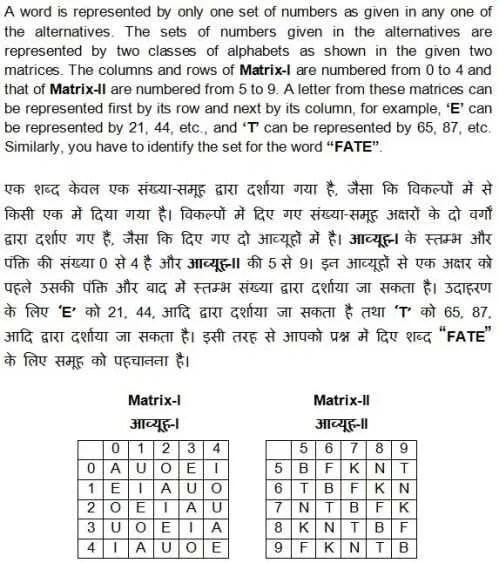## Matrix Coding in Reasoning

• Post author:

In Matrix Coding Questions of Reasoning, Normally, a letter from these matrices can be represented first by its row and next by its column. A word is represented by only one set of numbers given in any one of the alternatives. Concept and Questions for SSC CGL, CPO, CHSL, RRB and Competitive Exams in Hindi and English.

## Matrix Coding

Today, I will explain the Concept with Questions of Matrix Coding.

The sets of numbers given in the alternatives are represented by two classes of alphabets as in two matrices. The columns and rows of Matrix I are numbered – form 0 to 4 and that Matrix II are numbered from 5 to 9. A letter from these matrices can be represented first by its row and next by its column. A word is represented by only one set of numbers as given in any one of the options/ alternatives. You have to select correct option.

### Example

A word is represented by only one set of numbers as given in any one of the alternatives. The sets of numbers given in the alternatives are represented by two classes of alphabets as in two matrices given below. The columns and rows of Matrix I are numbered – form 0 to 4 and that Matrix II are numbered from 5 to 9. A letter from these matrices can be represented first by its row and next by its column, e.g., ‘A’ can be represented by 01, 14, etc. and E can be represented by 55, 66 etc. Similarly, you have to identify the set for the word ‘BEST’.

Matrix – I

Matrix – II

#### Explanation and Solution

Read the instructions and Look at the position of A in Matrix (Colored Red for easy identification)

A is at following 3 Positions

Row -0, Column -1 (01), Row-1, Column -4 (14) and Row 3 Column 0 (30)

Similar Manner E position is (Row, Column) – (55), (66), (77), (88)

Now we have to look for the code for BEST from the available options.

B can be represented (57), (65),(78) and (96)
That Means (a) and (d) are incorrect. and correct answer will be from (c) and (d)

E can be represented by (55), (66), (77), (88)
Only (c) match the condition from (b) and (c)

### Matrix Practice Questions

0

Matrix Coding

1 / 4

Matrix2 / 4

Matrix3 / 4

Matrix –   FATE4 / 4

Matrix Coding